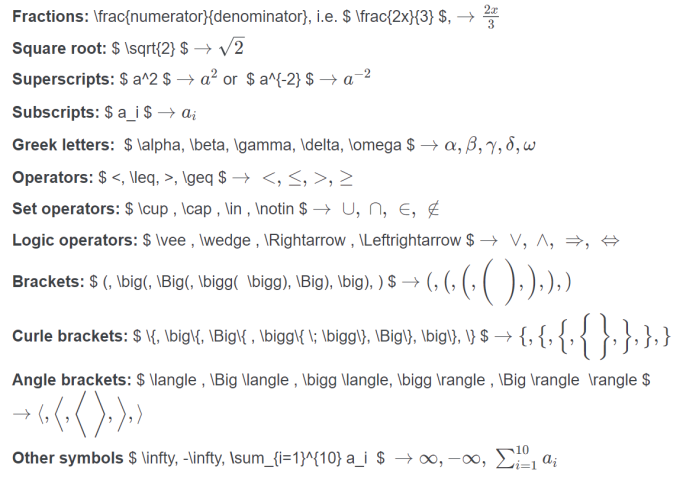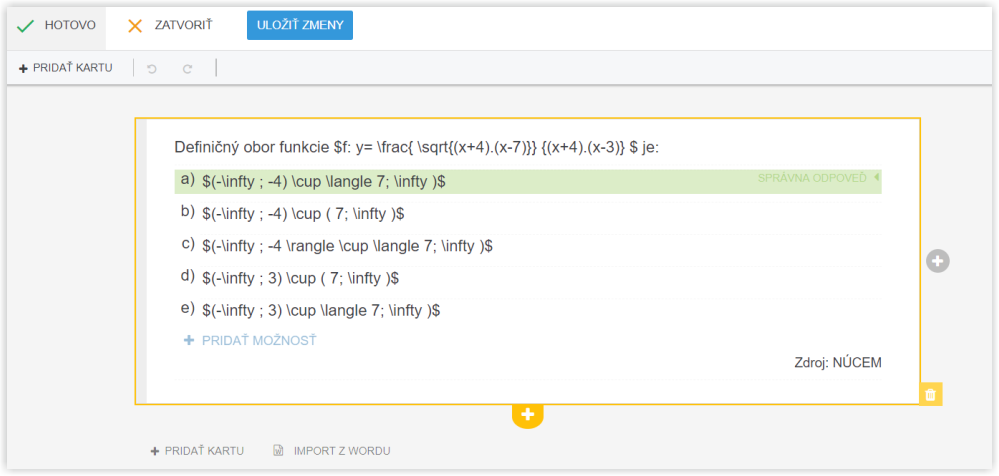EN# How to enter mathematical symbols in questions?

To enter mathematical expressions, LaTeX syntax is used. Instructions for use of LaTeX can be found on the Internet, for example here:

• KaTeX supported symbols

Mathematical expressions to be displayed in a line between the text must be enclosed in two dollars, for instance: $x^3 = 9$.

Expressions (formulas) to be displayed on a separate line must be enclosed in two dollars $$...$$.

There are examples of often used expressions:Example of one question card:The dollar sign is used for entering mathematical expressions. If you need to use the dollar sign in different situation e.g. excel expressions insert a backslash before the dollar sign: \\$. The system will recognize this sign and it will not consider the dollar sign as the beginning of a mathematical expression.